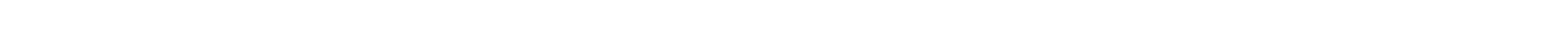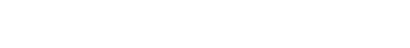## JavaScript Tutorial

In programming, there are many times when you need to do the same task much time. One way to do is to write the same type of statement many times or the more efficient way is to use a loop. JavaScript has many different types of loops. We will look into while and do…while here.The while loopIt is one of the most basic loops. The statements inside the loop will execute until the condition is true. The moment the condition becomes false, the loop will be exited.var counter = 10; while(counter > 0) {  console.log("Counter is ", counter);  counter--; }//Output -//Counter is 10  //Counter is 9  //Counter is 8  //Counter is 7  //Counter is 6  //Counter is 5  //Counter is 4  //Counter is 3  //Counter is 2  //Counter is 1In the above example, we have a variable counter set to 10. In the while loop condition we are checking whether counter > 0.Then inside the while, we have two statements. First once prints the value of the counter to the console and the second one decrements the counter value by 1.The first time it will print Counter is 10 and then decrement the value to 9. Now, the while loop condition will check whether 9>0 and it’s true, so it will print Counter is 9.This printing will go till Counter is 1. Now the decrement statement will make a counter to 0. The while loop condition will now check 0>0, which is false and the while loop will be exited.The do…while loopThe do…while loop is quite similar to the while loop except that the condition check happens at the end of the loop. That means the loop will be executed at least once, even if the condition is false.Let's check the below example.var counter = 10; while(counter > 10) {  console.log("Counter is ", counter);  counter--; } do{  console.log("Counter inside do-while is ", counter);  counter--; }while(counter > 10); // Counter inside do-while is 10Here, we have both a while loop and a do…while loop. In both the loops, the condition will result in false because the counter is 10 and we are checking whether 10 > 10.The while loop is exited the moment the condition is false and its console log is not printed. In the case of do…while, the console log is printed once and then the condition is checked and the loop is exited.

# while and do…while loops

In programming, there are many times when you need to do the same task much time. One way to do is to write the same type of statement many times or the more efficient way is to use a loop. JavaScript has many different types of loops. We will look into while and do…while here.

## The while loop

It is one of the most basic loops. The statements inside the loop will execute until the condition is true. The moment the condition becomes false, the loop will be exited.

var counter = 10;
while(counter > 0) {
console.log("Counter is ", counter);
counter--;
}

//Output -

//Counter is 10
//Counter is 9
//Counter is 8
//Counter is 7
//Counter is 6
//Counter is 5
//Counter is 4
//Counter is 3
//Counter is 2
//Counter is 1

In the above example, we have a variable counter set to 10. In the while loop condition we are checking whether counter > 0.
Then inside the while, we have two statements. First once prints the value of the counter to the console and the second one decrements the counter value by 1.
The first time it will print Counter is 10 and then decrement the value to 9. Now, the while loop condition will check whether 9>0 and it’s true, so it will print Counter is 9.
This printing will go till Counter is 1. Now the decrement statement will make a counter to 0. The while loop condition will now check 0>0, which is false and the while loop will be exited.

## The do…while loop

The do…while loop is quite similar to the while loop except that the condition check happens at the end of the loop. That means the loop will be executed at least once, even if the condition is false.

Let's check the below example.

var counter = 10;
while(counter > 10) {
console.log("Counter is ", counter);
counter--;
}
do{
console.log("Counter inside do-while is ", counter);
counter--;
}while(counter > 10);
// Counter inside do-while is 10

Here, we have both a while loop and a do…while loop. In both the loops, the condition will result in false because the counter is 10 and we are checking whether 10 > 10.
The while loop is exited the moment the condition is false and its console log is not printed. In the case of do…while, the console log is printed once and then the condition is checked and the loop is exited.#### Janani

I have learned many things from this article. It is beneficial for me. Thank you!#### Nilesh Chakrabarty

Nice example for beginners.. I m a beginner so this is very helpful for me ... so plz give this type of beginners example..#### michael

This is a great introduction to variables in JavaScript! As a beginner to JavaScript, I found this guide very helpful in understanding the basics of variables and how they are used in JavaScript.#### qtsinfo it

Thanks for sharing the information, it is very helpful, I hope to get more such beautiful blogs from you.#### knowledge-wisdom

You have shared great information with me i appreciate your work!

## Node JS Tutorial

Build various types of web applications,command-line application,etc.
11185
Node JS Tutorial

Build various types of web applications,command-line application,etc....